# Coded Binary Numbers Online Quiz

Following quiz provides Multiple Choice Questions (MCQs) related to Coded Binary Numbers. You will have to read all the given answers and click over the correct answer. If you are not sure about the answer then you can check the answer using Show Answer button. You can use Next Quiz button to check new set of questions in the quiz.Go through the following information and answer the question carefully.

In a certain code, the symbol for 0 (zero) is @ and for 1 is $. There is no symbol for rest of the numbers. Numbers greater than 1 are needed to be depicted using the two given symbols. Left shifting of 1 doubles its value each time. Study the following example. '0' is depicted as @ '1' is depicted as$

'2' is depicted as $@ '3' is depicted as $$'4' is depicted as @@ and so on. Q 1 - Which of the following numbers will be represented by$$@$$? Options : A - 18 B - 17 C - 23 D - 27 E - None of these ### Answer : D ### Explanation$$@$$= 11011 = 1 x 24 + 1 x 23 + 0 x 22 + 1 x 21 + 0 x 20 = 16 + 8 + 0 + 2 + 1 = 27 Go through the following information and answer the question carefully. In a certain code, the symbol for 0 (zero) is > and for 1 is =. There is no symbol for rest of the numbers. Numbers greater than 1 are needed to be depicted using the two given symbols. Left shifting of 1 doubles its value each time. Study the following example. '0' is depicted as > '1'is depicted as = '2' is depicted as = > '3' is depicted as = = '4' is depicted as = >> and so on Q 2 - Which of the following will represent 9? Options : A - = >> = > B - > = >> C - = >>> D - = = >> E - None of these ### Answer : E ### Explanation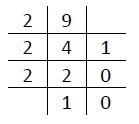(9)10 = (1001)2 ⇒ (= >>=) Go through the following information and answer the question carefully. In a certain code, the symbol for 0 is • and for 1 is #. There is no symbol for rest of the numbers. Numbers greater than 1 are needed to be depicted using the two given symbols. Left shifting of 1 doubles its value each time. Study the following example. 0 is written as • 1 is written as # 2 is written as at 3 is written as ## 4 is written as #•• and so on. Q 3 - Which of the following will represent 111? Options : A - ##•##### B - ####•## C - ##•#### D - ###•#### E - None of these ### Answer : C ### Explanation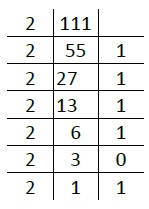∴ (111)10 = (1101111)2 = ## • #### Go through the following information and answer the question carefully. In a certain code, the symbol for 0 is ) and for 1 is Δ. There is no symbol for rest of the numbers. Numbers greater than 1 are needed to be depicted using the two given symbols. Left shifting of 1 doubles its value each time. Study the following example. 0 is written as ) 1 is written as Δ 2 is written as Δ) 3 is written as Δ Δ 4 is written as Δ) ) and so on. Q 4 - Ram does a work in fifteen days. Shyam does the same work in twelve days. Ram left the work after working for five days. Then Shyam took over. Shyam will finish the rest of the work in X days. X will be represented by Options : A - Δ))) B - Δ)) C - Δ)Δ) D - Δ))Δ E - None of these ### Answer : A ### Explanation In 1 day Ram does$\frac{1}{15}$work$\therefore$In 5 days Ram does$\frac{1}{3}$work$\therefore$Work left for Shyam =$\left ( 1 - \frac{1}{3} \right ) = \frac{2}{3}\because$Shyam does one work in 12 days$\therefore$Shyam does$\frac{2}{3}$work in$\frac{12 x 2}{3}\$ = 8 days

Now,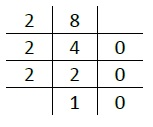(8)10 (1000)2 = Δ***

Go through following information and answer the question accordingly.

In a certain code, the symbol for 0 is - and for 1 is (. There is no symbol for rest of the numbers. Numbers greater than 1 are needed to be depicted using the two given symbols. Left shifting of 1 doubles its value each time. Study the following example.

'0' is depicted as -

'1' is depicted as (

'2' is depicted as (-

'3' is depicted as ((

'4' is depicted as (-- and so on

Q 5 - Which of the following will represent 11?

Options :

A - (-((

B - -(--

C - ((((

D - (-(-(

E - None of these

### Explanation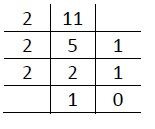So (11)10 = (1011)2 = (-((

Go through the following information and answer questions accordingly.

In a certain code, the symbol 0 is written as + and for 1 is *. There is no symbol for rest of the numbers. Numbers greater than 1 are needed to be written using the two given symbols. Left shifting of 1 doubles its value each time. Study the following example.

0 is written as +

1 is written as *

2 is written as *+

3 is written as **

4 is written as *++

Q 6 - Which of the following numbers will be represented by +****?

Options :

A - 17

B - 16

C - 18

D - 15

### Explanation

The number is +**** = (01111)2 = (15)10

Go through the following information and answer the question accordingly.

In a certain code, the symbol for 0 is * and for 1 is Δ . There is no symbol for rest of the numbers. Numbers greater than 1 are needed to be written using the two given symbols. Left shifting of 1 doubles its value each time. Study the following example.

0 is written as *

1 is written as Δ

2 is written as Δ*

3 is written as Δ Δ

4 is written as Δ** and so on.

Q 7 - Which of the following will represent 52?

Options :

A - Δ**Δ**

B - Δ*Δ Δ Δ

C - Δ*****

D - Δ Δ*Δ**

E - None of these

### Explanation

The decimal to binary conversions will be (52)10 = (110100)2

Try to solve the questions by deep analyzing the given information.

In a certain code, the symbol for 0 (zero) is Δ and for 1 is +. There is no symbol for rest of the numbers. Numbers greater than 1 are needed to be written using the two given symbols. Left shifting of 1 doubles its value each time. Study the following example.

'0' is written as Δ

'1' is written as +

'2' is written as +Δ

'3' is written as ++

'4' is written as +Δ Δand so on.

Q 8 - Which of the following will represent 7 x (l + 4 ÷ 2)?

Options :

A - +Δ+Δ+

B - ++Δ+Δ

C - +Δ Δ++

D - +Δ Δ+Δ

E - None of these

### Explanation

7 x (1 + 4 ÷ 2) = 7 x (1 + 2) = 7 x 3 = 21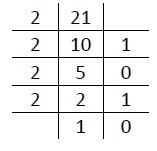(21)10 = (10101)2 = +Δ+Δ+

Try to solve the questions by deep analyzing the given information.

In a certain code, the symbol for 0 is + and for 1 is *. There is no symbol for rest of the numbers. Numbers greater than 1 are needed to be written using the two given symbols. Left shifting of 1 doubles its value each time. Study the following example.

0 is written as +

'1' is written as *

2 is written as *+

3 is written as **

4 is written as *++ and so on

Q 9 - Which of the following will represent 10?

Options :

A - *+*+

B - ****

C - ++++

D - +*+*

### Explanation

After dividing 10 with 2, we can get remainders as 1010 which means the answer is *+*+.

Try to solve the questions by deep analyzing the given information.

In a certain code, the symbol for 0 is ! and for 1 is #. There is no symbol for rest of the numbers. Numbers greater than 1 are needed to be written using the two given symbols. Left shifting of 1 doubles its value each time. Study the following example.

0 is written as !

1 is written as #

2 is written as #!

3 is written as ##

4 is written as #!! and so on

Q 10 - Which of the following will represent 4 + 2 x 2 x 2 + 3 x 3 + 2 x 3?

Options :

A - ##!##

B - #!#!#

C - !#!#!

D - #!!##